Courses

# Test: The P-Block Elements 1 - From Past 28 Years Questions

## 20 Questions MCQ Test Chemistry 28 Years Past year papers for NEET/AIPMT Class 12 | Test: The P-Block Elements 1 - From Past 28 Years Questions

Description
This mock test of Test: The P-Block Elements 1 - From Past 28 Years Questions for Class 12 helps you for every Class 12 entrance exam. This contains 20 Multiple Choice Questions for Class 12 Test: The P-Block Elements 1 - From Past 28 Years Questions (mcq) to study with solutions a complete question bank. The solved questions answers in this Test: The P-Block Elements 1 - From Past 28 Years Questions quiz give you a good mix of easy questions and tough questions. Class 12 students definitely take this Test: The P-Block Elements 1 - From Past 28 Years Questions exercise for a better result in the exam. You can find other Test: The P-Block Elements 1 - From Past 28 Years Questions extra questions, long questions & short questions for Class 12 on EduRev as well by searching above.
QUESTION: 1

### Which one of the  following orders is not in accordance with the property stated against is ? 

Solution:

Bond dissociation energy of fluorine is less because of its small size and repulsion between electrons of two atoms. So option (c) is wrong order. The correct order is [Cl2 > Br2 > F2 > I2]

QUESTION: 2

### Which one of the following orders correctly represents the increasing acid strengths of the given acids? 

Solution: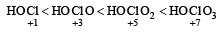As the oxidation number of the central atom increases, strength of acid also increases.

*Multiple options can be correct
QUESTION: 3

### Which one of the following  arrangements does not give the correct picture of the trends indicated against it ? 

Solution:

From the given options we find option (a) is correct. The oxidising power of halogens follow the order F2 > Cl2 > Br2 > I2 . Option (b) is incorrect because it in not the correct order of electron gain enthalpy of halogens. The correct order is Cl2 > F2 > Br2 > I2 . The low value of F2 than Cl2 is due to its small size. Option (c) is incorrect. The correct order of bond dissociation energies of halogens is Cl2 > Br2 > F2 > I2 . Option (d) is correct. It is the correct order of electronegativity values of halogens. Thus option (b) and (c) are incorrect.

QUESTION: 4

In the case of alkali metals, the covalent character decreases in the order: 

Solution:

MI > MBr > MCl > MF. As the size of the anion decreases covalency decreases.

QUESTION: 5

Among the following which is the strongest oxidising agent? 

Solution:

Standard reduction potential of halogens are positive and decreases from fluorine to iodine. Therefore halogens act as strong oxidising agent and their oxidising power decreases from fluorine to iodine.

QUESTION: 6

The correct order of increasing bond angles in the following species are : 

Solution:

The correct order of increasing bond angle is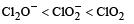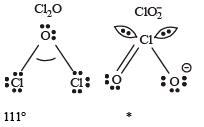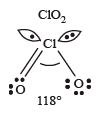* In ClO2there are 2 lone pairs of electrons present on the central chlorine atom. Therefore the bond angle in ClO2 is less than 118° which is the bond angle in ClO2 which has less number of electrons on chlorine.

QUESTION: 7

Oxidation states of P in H4 P2O5 , H4 P2O6 , and H4 P2O7 , are respectively: 

Solution:

The correct answer is Option D.

H4P2O5 ;
​4+2x−10=0
​2x=6
x= +3

H4P2O6
4+2x−12=0
2x=8
x= +4

H4P2O7
4+2x−14=0
2x=10
x= +5

QUESTION: 8

Which one of the following compounds is a peroxide ? 

Solution: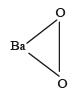QUESTION: 9

Match List - I (substan ces) with List - II (processes) employed in the manufacture of the substances and select the correct option.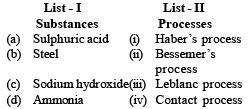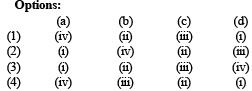Solution:

(a) Sulphuric acid (iv) Contact process
(b) Steel (ii) Bessemer ’s process
(c) Sodium hydroxide(iii) Leblanc process
(d) Ammonia (i) Haber ’s process
∴ Correct choice : (a)

QUESTION: 10

How many bridging oxygen atoms are present in P4O10? 

Solution: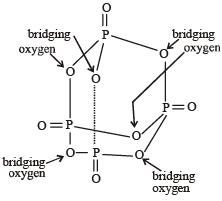∴ Correct choice : (b)

QUESTION: 11

Which of the following structures is the most preferred and hence of lowest energy for SO3 ? [2011 M]

Solution:

Formal charges help in the selection of the lowest energy structure from a number of possible Lewis structures for a given compound. The lowest energy structure means the structure with the smallest formal charge on each atom of the compound. A Lewis dot structure is preferable when all formal charges are zero.

QUESTION: 12

Which of the following oxide is amphoteric ?

Solution:

SnO2 is an amphoteric oxide because it reacts with acids as well as bases to form corresponding salts.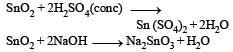QUESTION: 13

In which of the following compounds, nitrogen exhibits highest oxidation state ? 

Solution: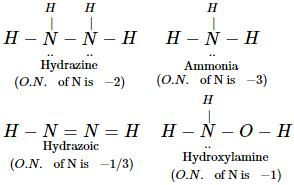Thus, nitrogen exhibits highest oxidation state in N3H
Thus question can also be credited to redox reactions

QUESTION: 14

Which of the following statements is not valid for oxoacids of phosphorus? 

Solution: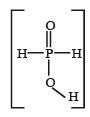Hypophosphorous acid

(H3PO2) is a monobasic acid. i.e., it has only one ionisable hydrogen atom or one OH is present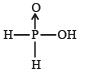QUESTION: 15

Sulphur trioxide can be obtained by which of the following reaction : 

Solution: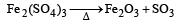QUESTION: 16

Which is the strongest acid in the following : [NEET 2013]

Solution:

HClO4 is the strongest acid amongst all because the oxidation state or Cl is maximum (+7).

QUESTION: 17

Which of the following does not give oxygen on heating? [NEET 2013]

Solution: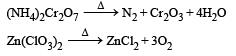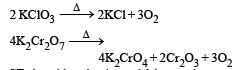QUESTION: 18

Which of the following is a polar molecule ? [NEET 2013]

Solution:

SF4 has 4 bond pairs and 1 lone pair of electrons, sp3d hybridisation leads to irregular shape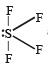and resultant

μ ≠ 0.

QUESTION: 19

Identify the incorrect statement, regarding the molecule XeO4: [NEET Kar. 2013]

Solution: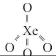QUESTION: 20

In which of the following arrangements the given sequence is not strictly according to the property indicated against it ? [2012 M]

Solution:

If acidic nature is high, Ka is high and pka is low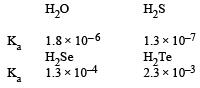since pka = – log ka
Hence the order of pka will be H2O > H2S > H2Se > H2Te